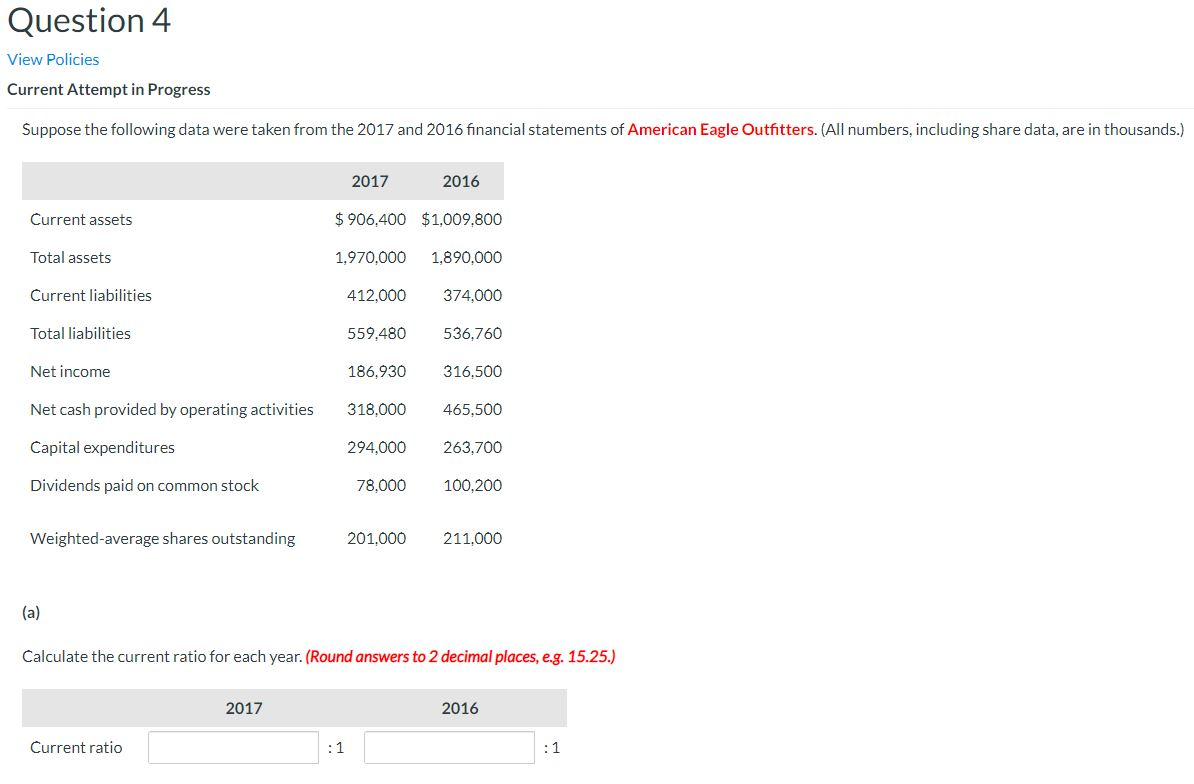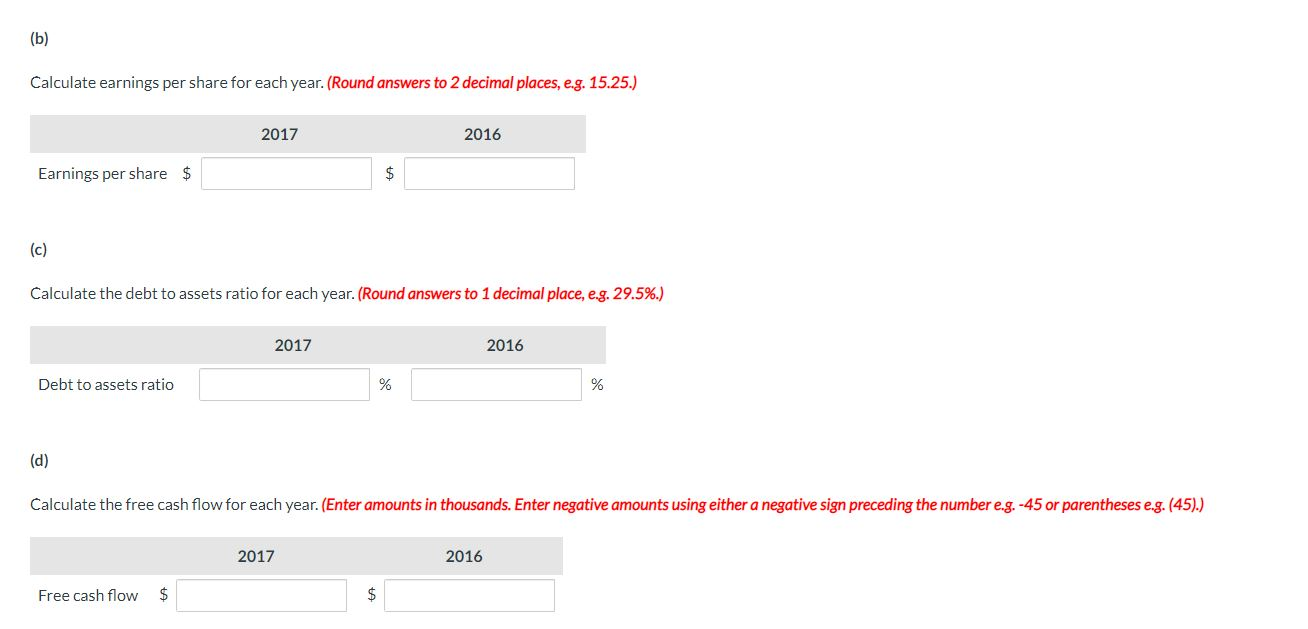Home / Answered Questions / Other / question-4-view-policies-current-attempt-in-progress-suppose-the-following-data-were-taken-from-the--aw520

# (Solved): Question 4 View Policies Current Attempt In Progress Suppose The Following Data Were Taken From The ...Question 4 View Policies Current Attempt in Progress Suppose the following data were taken from the 2017 and 2016 financial statements of American Eagle Outfitters. (All numbers, including share data, are in thousands.) 2017 2016 Current assets \$906,400 \$1,009,800 1,970,000 1,890,000 Total assets Current liabilities 412,000 374,000 Total liabilities 559,480 536,760 Net income 186,930 316,500 Net cash provided by operating activities 465,500 318,000 294,000 Capital expenditures 263,700 Dividends paid on common stock 78,000 100,200 Weighted average shares outstanding 201,000 211,000 (a) Calculate the current ratio for each year. (Round answers to 2 decimal places, e.g. 15.25.) 2017 2016 Current ratio Calculate earnings per share for each year. (Round answers to 2 decimal places, e.g. 15.25.) 2017 2016 Earnings per share \$ Calculate the debt to assets ratio for each year. (Round answers to 1 decimal place, e.g. 29.5%.) 2017 2016 Debt to assets ratio (d) Calculate the free cash flow for each year. (Enter amounts in thousands. Enter negative amounts using either a negative sign preceding the number e.g. -45 or parentheses e.g. (45).) 2017 2016 Free cash flow \$

We have an Answer from Expert